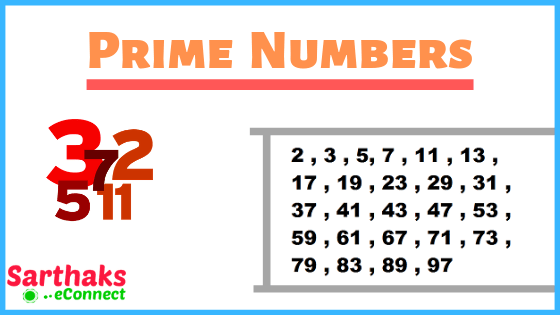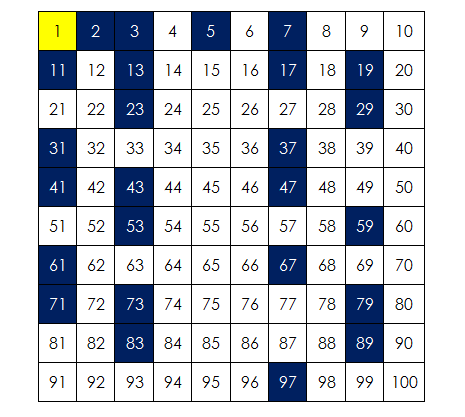# Prime Numbers:

Numbers which can be divided by 1 and itself only are called prime numbers.
Let’s take an example of 7 which can only be divided by 1 and 7 only. Thus 7 is a prime number.

• Prime numbers are positive natural numbers always greater than 1.
• No any number can divide any prime number without leaving any remainder.
• Any number except 0 and 1 can only be either prime number or composite number.

## Prime Numbers list:

Euclid around 300 BC said that there are infinitely many prime numbers. However, we have provided prime numbers list up to 1000. Take a look on it.

 2 3 5 7 11 13 17 19 23 29 31 37 41 43 47 53 59 61 67 71 73 79 83 89 97 101 103 107 109 113 127 131 137 139 149 151 157 163 167 173 179 181 191 193 197 199 211 223 227 229 233 239 241 251 257 263 269 271 277 281 283 293 307 311 313 317 331 337 347 349 353 359 367 373 379 383 389 397 401 409 419 421 431 433 439 443 449 457 461 463 467 479 487 491 499 503 509 521 523 541 547 557 563 569 571 577 587 593 599 601 607 613 617 619 631 641 643 647 653 659 661 673 677 683 691 701 709 719 727 733 739 743 751 757 761 769 773 787 797 809 811 821 823 827 829 839 853 857 859 863 877 881 883 887 907 911 919 929 937 941 947 953 967 971 977 983 991 997

By this prime numbers chart you can analyze its importance in arithmetic.

## Prime Numbers From 1 to 100:

There is no common pattern to describe every prime number. The list of prime numbers from 1 to 100 is provided below:

 2 3 5 7 11 13 17 19 23 29 31 37 41 43 47 53 59 61 67 71 73 79 83 89 97

Further explained as:## Even Prime Number:

2 is the one and only even prime number.
As we know from the definition that prime numbers should have factors one and itself only. So, when we notice even numbers we see that 2 is the only even number which has its factor 1 and itself. So, 2 is the only even prime number. Let’s confirm it by taking another example of even number 8. Factors of 8 are 1, 2, 4, 8. Thus at any cost, it can’t be prime number as there are more numbers which can divide 8.

## How to find Prime numbers?

There is a simple procedure to find prime numbers. However, you have to follow some steps which are below:
Let’s suppose any number ‘K’ and we have to find whether it is prime or not.

Step 1:
Do its trial square root and take a whole number nearly its square root (√K = L).

Step 2:
Then we will test that if the number can be divisible by any of the prime number less than given number (L). If not divisible then it is a prime number otherwise it is not a prime number.

Example:
Let’s check whether 281 is prime or not. At first, we take its approx nearest square root which is 17.

The prime numbers less than 17 are 2, 3, 5, 7, 11, 13, 17.
281 is not divisible by any of the prime numbers so is a prime number.

## Is 0 a prime number?

0 is not a prime number.
According to the definition of prime numbers that it should be the positive integer and always be greater than 1. 0 doesn’t follow the rule so it’s not a prime number.]

## Is 1 a prime number?

1 is not a prime number.
According to the definition of prime number, it must have two factors 1 and that number itself but 1 has only one factor so 1 is not a prime number.

## Is 2 a prime number?

2 is a prime number. Also, it is the only even prime number.
It correctly follows the definition of prime numbers as it has only two factors 1 and 2 itself so 2 is a prime number.

Prime number is a part of real number. Further it comes in natural number.